×#### Thank you for registering.

One of our academic counsellors will contact you within 1 working day.

Click to Chat

1800-1023-196

+91-120-4616500

CART 0

• 0

MY CART (5)

Use Coupon: CART20 and get 20% off on all online Study Material

ITEM
DETAILS
MRP
DISCOUNT
FINAL PRICE
Total Price: Rs.

There are no items in this cart.
Continue Shopping• Complete JEE Main/Advanced Course and Test Series
• OFFERED PRICE: Rs. 15,900
• View Details

```Revision Notes on Areas Related to Circles

Perimeter and Area of a Circle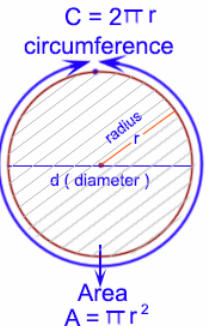The perimeter of all the plain figures is the outer boundary of the figure. Likewise, the outer boundary of the circle is the perimeter of the circle. The perimeter of circle is also called the Circumference of the Circle.

Circumference = 2πr = πd (π = 22/7)

r = Radius and d = 2r

The area is the region enclosed by the circumference.

Area of the circle = πr2

Example

If a pizza is cut in such a way that it divides into 8 equal parts as shown in the figure, then what is the area of each piece of the pizza? The radius of the circle shaped pizza is 7 cm.Solution

The pizza is divided into 8 equal parts, so the area of each piece is equal.

Area of 1 piece = 1/8 of area of circle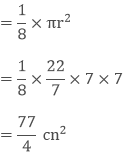Perimeter and Area of the Semi-circle

The perimeter of the semi-circle is half of the circumference of the given circle plus the length of diameter as the perimeter is the outer boundary of the figure.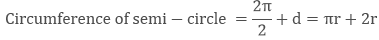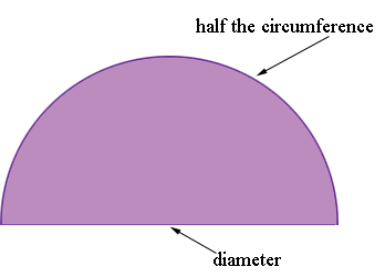Area of the semi-circle is just half of the area of the circle.Area of a RingArea of the ring i.e. the coloured part in the above figure is calculated by subtracting the area of the inner circle from the area of the bigger circle.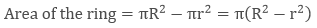Where, R = radius of outer circle

r = radius of inner circle

Areas of Sectors of a Circle

The area formed by an arc and the two radii joining the endpoints of the arc is called Sector.Minor Sector

The area including ∠AOB with point C is called Minor Sector. So OACB is the minor sector. ∠AOB is the angle of the minor sector.Major Sector

The area including ∠AOB with point D is called the Major Sector. So OADB is the major sector. The angle of the major sector is 360° – ∠AOB.

Area of Major Sector = πr2 -  Area of the Minor Sector

Remark: Area of Minor Sector + Area of Major Sector = Area of the Circle

Length of an Arc of a Sector of Angle θ

An arc is the piece of the circumference of the circle so an arc can be calculated as the θ part of the circumference.Areas of Segments of the Circle

The area made by an arc and a chord is called the Segment of the Circle.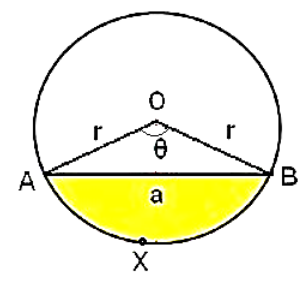Minor Segment

The area made by chord AB and arc X is the minor segment. The area of the minor segment can be calculated by

Area of Minor Segment = Area of Minor Sector – Area of ∆ABO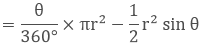Major Segment

The other part of the circle except for the area of the minor segment is called a Major Segment.

Area of Major Segment = πr2 -  Area of Minor Segment

Remark: Area of major segment + Area of minor segment = Area of circle

Areas of Combinations of Plane Figures

As we know how to calculate the area of different shapes, so we can find the area of the figures which are made with the combination of different figures.

Example

Find the area of the colored part if the given triangle is equilateral and its area is 17320.5 cm2. Three circles are made by taking the vertex of the triangles as the centre of the circle and the radius of the circle is the half of the length of the side of the triangle. (π = 3.14 and √3 = 1.73205)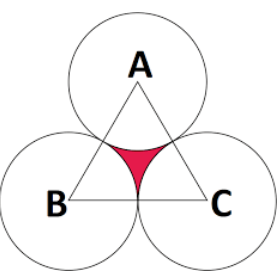Solution

Given

ABC is an equilateral triangle, so ∠A, ∠B, ∠C = 60°

Hence the three sectors are equal, of angle 60°.

Required

To find the area of the shaded region.

Area of shaded region =Area of ∆ABC – Area of 3 sectors

Area of ∆ABC = 17320.5 cm2Side = 200 cm

As the radius of the circle is half of the length of the triangle, soArea of 3 Sectors = 3 × 15700/3 cm2 cm2

Area of shaded region = Area of ∆ABC – Area of 3 sectors

= 17320.5 - 15700 cm2

= 1620.5 cm2

Example

Find the area of the shaded part, if the side of the square is 8 cm and the 44 cm.Solution

Required region = Area of circle – Area of square

= πr2 – (side)2

Circumference of circle = 2πr = 44Radius of the circle = 7 cm

Area of circle = πr2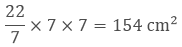Area of square = (side) 2 = (8)2 = 64 cm2

Area of shaded region = Area of circle – Area of square

= 154 cm2 - 64 cm2

= 90 cm2
```### Course Features

• 728 Video Lectures
• Revision Notes
• Previous Year Papers
• Mind Map
• Study Planner
• NCERT Solutions
• Discussion Forum
• Test paper with Video Solution